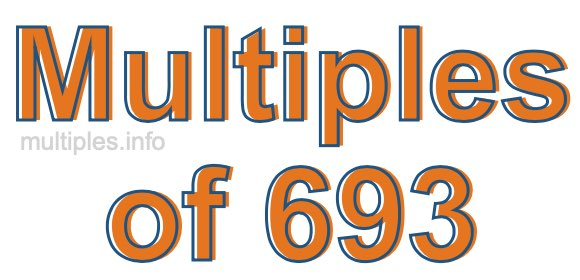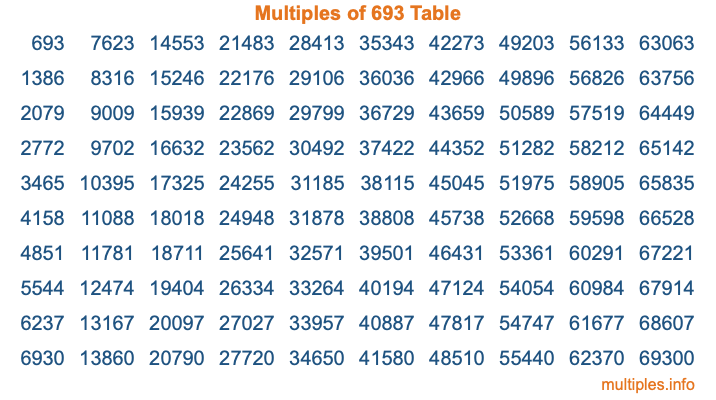Multiples of 693Welcome to the Multiples of 693 page. Here we will first teach you everything you will ever need to know about the multiples of 693, and then give you a study guide summary of everything we taught you to make sure you remember it all. Use this page to look up facts and learn information about the multiples of 693. This page will make you a multiples of six hundred ninety-three expert!

Definition of Multiples of 693
Multiples of 693 are all the numbers that when divided by 693 equal an integer. Each of the multiples of 693 are called a multiple. A multiple of 693 is created by multiplying 693 by an integer.

Therefore, to create a list of multiples of 693, you start with 1 multiplied by 693, then 2 multiplied by 693, then 3 multiplied by 693, and so on for as long as you want. Thus, the list of the first five multiples of 693 is 693, 1386, 2079, 2772, and 3465. To see a larger list of multiples of 693, see the printable image of Multiples of 693 further down on this page. We also have a category where you can choose any nth multiple of 693.

Multiples of 693 Checker
The Multiples of 693 Checker below checks to see if any number of your choice is a multiple of 693. In other words, it checks to see if there is any number (integer) that when multiplied by 693 will equal your number. To do that, we divide your number by 693. If the the quotient is an integer, then your number is a multiple of 693.

Is  a multiple of 693?

Least Common Multiple of 693 and ...
A Least Common Multiple (LCM) is the lowest multiple that two or more numbers have in common. This is also called the smallest common multiple or lowest common multiple and is useful to know when you are adding our subtracting fractions. Enter one or more numbers below (693 is already entered) to find the LCM.

Check out our LCM Calculator if you need more details about the Least Common Multiple or if you need the LCM for different numbers for adding and subtraction fractions.

nth Multiple of 693
As we stated above, 693 is the first multiple of 693, 1386 is the second multiple of 693, 2079 is the third multiple of 693, and so on. Enter a number below to find the nth multiple of 693.

th multiple of 693

Multiples of 693 vs Factors of 693
693 is a multiple of 693 and a factor of 693, but that is where the similarities end. All postive multiples of 693 are 693 or greater than 693. All positive factors of 693 are 693 or less than 693.

Below is the beginning list of multiples of 693 and the factors of 693 so you can compare:

Multiples of 693: 693, 1386, 2079, 2772, 3465, etc.

Factors of 693: 1, 3, 7, 9, 11, 21, 33, 63, 77, 99, 231, 693

As you can see, the multiples of 693 are all the numbers that you can divide by 693 to get a whole number. The factors of 693, on the other hand, are all the whole numbers that you can multiply by another whole number to get 693.

It's also interesting to note that if a number (x) is a factor of 693, then 693 will also be a multiple of that number (x).

Multiples of 693 vs Divisors of 693
The divisors of 693 are all the integers that 693 can be divided by evenly. Below is a list of the divisors of 693.

Divisors of 693: 1, 3, 7, 9, 11, 21, 33, 63, 77, 99, 231, 693

The interesting thing to note here is that if you take any multiple of 693 and divide it by a divisor of 693, you will see that the quotient is an integer.

Multiples of 693 Table
Below is an image of the first 100 multiples of 693 in a table. The table is in chronological order, column by column. The first column has the first ten multiples of 693, the second column has the next ten multiples of 693, and so on.The Multiples of 693 Table is also referred to as the 693 Times Table or Times Table of 693. You are welcome to print out our table for your studies.

Negative Multiples of 693
Although not often discussed or needed in math, it is worth mentioning that you can make a list of negative multiples of 693 by multiplying 693 by -1, then by -2, then by -3, and so on, to get the following list of negative multiples of 693:

-693, -1386, -2079, -2772, -3465, etc.

Multiples of 693 Summary
Below is a summary of important Multiples of 693 facts that we have discussed on this page. To retain the knowledge on this page, we recommend that you read through the summary and explain to yourself or a study partner why they hold true.

There are an infinite number of multiples of 693.

A multiple of 693 divided by 693 will equal a whole number.

693 divided by a factor of 693 equals a divisor of 693.

The nth multiple of 693 is n times 693.

The largest factor of 693 is equal to the first positive multiple of 693.

693 is a multiple of every factor of 693.

693 is a multiple of 693.

A multiple of 693 divided by a divisor of 693 equals an integer.

693 divided by a divisor of 693 equals a factor of 693.

Any integer times 693 will equal a multiple of 693.

Multiples of a Number
Here you can get the multiples of another number, all with the same attention to detail as we did for multiples of 693 on this page.

Multiples of
Multiples of 694
Did you find our page about multiples of six hundred ninety-three educational? Do you want more knowledge? Check out the multiples of the next number on our list!